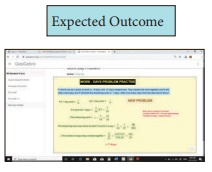Home | | Maths 8th Std | Summary

# Summary

8th Maths : Chapter 4 : Life Mathematics : Summary

SUMMARY

When the S.P is more than the C.P, then there is a gain or profit. Profit/Gain =S.P – C.P.

When the S.P is less than the C.P, then there is a loss. Loss = C.P – S.P.

The profit or loss is always calculated on the cost price.

Selling price = Marked price – Discount.

FormulaeWhen the interest is compounded annually but rate of interest differs year by year,where a, b and c are interest rates for the I, II and III years respectively.

When interest is compounded annually but time period is in fraction say a b/c years,C.I = A−P (Amount − Principal).

The simple interest and the compound interest remains the same for the first year or the first conversion period.

For 2 years, the difference in C.I and S.I is C.I − S.I =P (r/100)2.

For 3 years, the difference in C.I and S.I is C.I − S.I =x and y are said to vary directly if y = kx always, where k is called the proportionality constant and k > 0 assuming that y depends on x and so k = y/x.

x and y are said to vary inversely, if xy = k always, where k is called the proportionality constant and k > 0.

There will be problems which involve a chain of two or more variations in them. This is called as compound variation.

By finding the proportion, we can use the fact that the product of the extremes is equal to the product of the means to find the unknown (x) in the problem.

By using the formula= [ P1×D1×H1 ] / W1 = [ P2×D2×H2 ] / W2, we can find the unknown (x).

We can also find the unknown (x) by Multiplicative Factor Method.

If two persons X and Y can complete some work individually in a and b days, their one day’s work will be 1/a and 1/b respectively and X and Y together can complete the work in ab / a+b days.

ICT CORNERStep-1 Open the Browser type the URL Link given below (or) Scan the QR Code. GeoGebra work book named “LIFE MATHEMATICS” will open. Click on the worksheet named “Percentage”.

Step-2 In the given worksheet you can drag the red points E and F to change the Blue rectangle. Find the ratio of Blue with the whole by counting the squares and check the ratio and percentage.Go through the remaining worksheets given for this chapter

Life Mathematics:

https://www.geogebra.org/m/fqxbd7rz#chapter/409575 or Scan the QR Code.

*Pictures are indicatives only.

*If browser requires, allow Flash Player or Java Script to load the page.

ICT CORNERStep-1 Open the Browser type the URL Link given below (or) Scan the QR Code. GeoGebra work sheet named “8th Standard III term” will open. Select the work sheet named “Work Day Problem”

Step-2 Click on “ NEW PROBLEM”. Check the calculation and work out yourself.